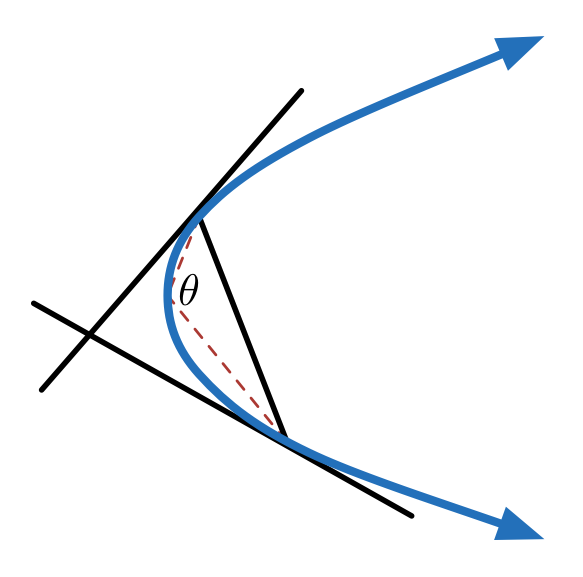# Two Points On A Parabola

Calculus Level 3Two points are chosen at a parabola, such that tangents at these points are perpendicular to each other . Let the angle subtended by the chord joining the points at the vertex of the parabola be $\theta$ . Find the value of $\theta_{max} + \theta_{min}$ in degrees.

×